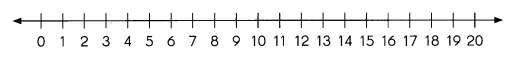# Math in Focus Grade 3 Chapter 6 Practice 1 Answer Key Multiplication Properties

Go through the Math in Focus Grade 3 Workbook Answer Key Chapter 6 Practice 1 Multiplication Properties to finish your assignments.

## Math in Focus Grade 3 Chapter 6 Practice 1 Answer Key Multiplication Properties

Look at each number line. Write the multiplication fact.

Question 1.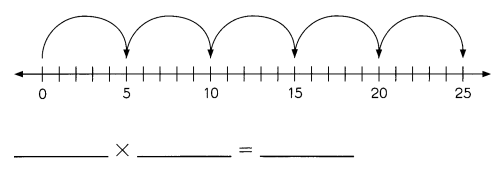5×5 = 25.

Explanation:
In the number line, we can see that 5 jumps of 5. So the multiplication fact is 5×5 which is 25.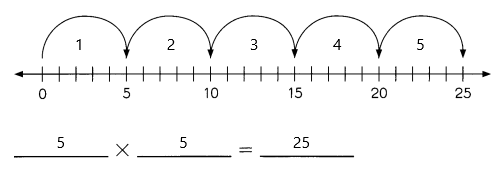Question 2.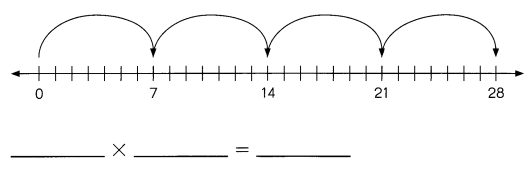4×7 = 28.

Explanation:
In the number line, we can see that 4 jumps of 7. So the multiplication fact is 4×7 which is 28.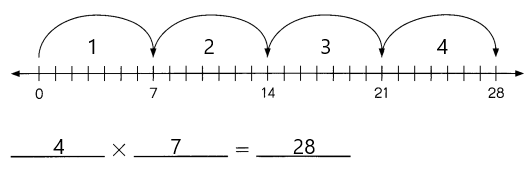Question 3.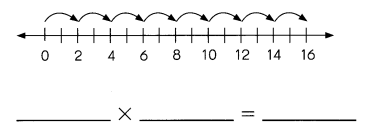2×8 = 16.

Explanation:
In the number line, we can see that 2 jumps of 8. So the multiplication fact is 2×8 which is 16.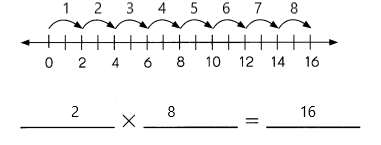Complete each multiplication fact. Then show on each number line.

Question 4.
4 × 5 = ____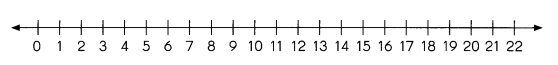4 × 5 = 20.

Explanation:
We will represent in the number line by 4 jumps of 5 for 4 × 5 which is 20.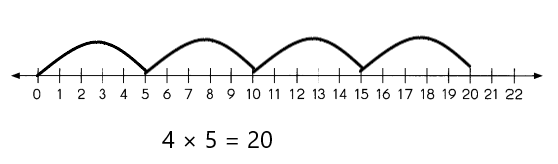Question 5.
5 × 3 = ____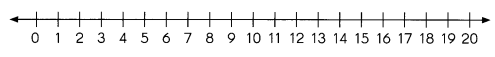5 × 3 = 15.

Explanation:
We will represent in the number line by 5 jumps of 3 for 5 × 3 which is 15.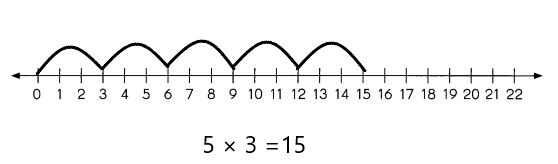Look at the dot paper. Write the multiplication fact.

Example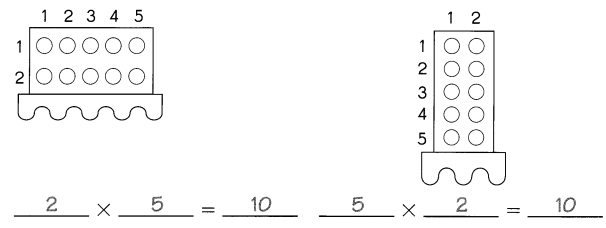Question 6.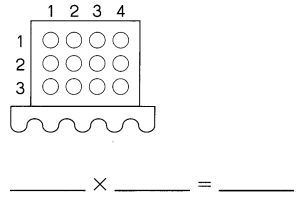3 × 4 = 12.

Explanation:
The multiplication between 3 and 4 is 3 × 4 which is 12.

Question 7.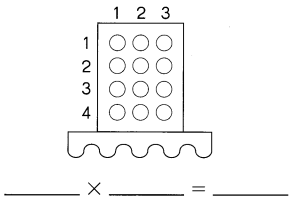2 × 3 = 6.

Explanation:
The multiplication between 2 and 3 is 2 × 3 which is 6.

Look at the dot paper. Write the multiplication fact.

Question 7.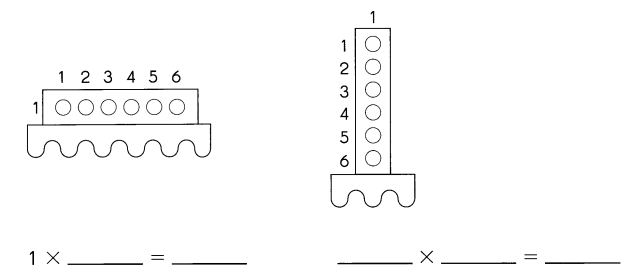1 × 5 = 5.

Explanation:
The multiplication between 1 and 5 is 1 × 5 which is 5.

Question 8.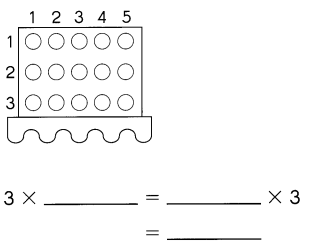3 × 5 = 5 × 3 = 15.

Explanation:
The multiplication between 3 and 5 is 3 × 5 which is 15.

Complete the multiplication fact. Then show on each number line.

Question 9.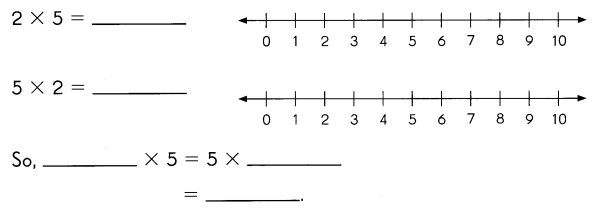Fill in the missing numbers.

Question 10.
2 × __ = 0 × ___
= ____
2 × 0 = 0 × 2 = 0.

Explanation:
Here, if we multiply 0 with anything we will get the product as 0. So
2 × 0 = 0 × 2
= 0.
So the missing letter is 0.

Question 11.
0 × ___ = 4 × ___
= _____
0 × 4 = 4 × 0 =0.

Explanation:
Here, if we multiply 0 with anything we will get the product as 0. So
0 × 4 = 4 × 0
= 0.
So the missing letter is 4.

Question 12.
5 × ___ = 1 × ___
= ___
5 × 1 = 1 × 5 = 5.

Explanation:
Here the missing letter is 1, as
5 × 1 = 1 × 5
= 5.
So the missing letter is 1.

Question 13.
1 × ___ = 10 × ___
= ____
1 × 10 = 10 × 1 = 10.

Explanation:
Here the missing letter is 1, as
1 × 10 = 10 × 1
= 10.
So the missing letter is 1.

Complete each multiplication fact. Then show on the number line.

Example
2 × 3 × 3 = ?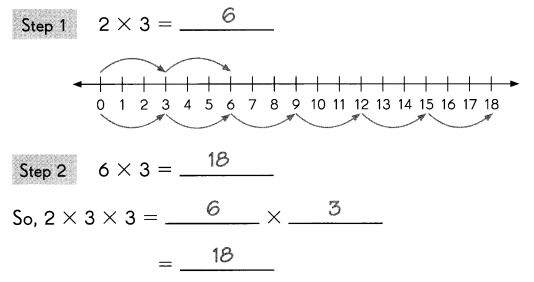Question 14.
2 × 4 × 2 = ___ × ____
= _____